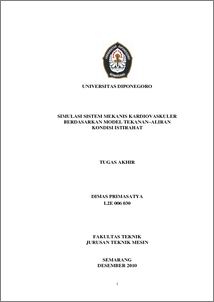# Simulasi Sistem Mekanis Kardiovaskuler Berdasarkan Model Tekanan - Aliran Kondisi Istirahat

PRIMASATYA, Dimas and HARYANTO, Ismoyo (2011) Simulasi Sistem Mekanis Kardiovaskuler Berdasarkan Model Tekanan - Aliran Kondisi Istirahat. Undergraduate thesis, Mechanical Engineering Departement of Diponegoro University.Preview
PDF
1018Kb

Official URL: http://www.mesin.ft.undip.ac.id/perpustakaan/

## Abstract

Non-invasive measurement method has made rapid developments in the field of biomedical engineering. One of research is impedance cardiography (ICG), which provide information of pulsation basic. By knowing this kind of measurement technique, it will assist inspection of the patient's physiological condition with cardiovascular system. This research is aimed to determine the mechanical characteristics of the cardiovascular system in the human body such as a wave graph of pressure, flow, and volume, based on pressure–flow model in rest condition, and analyze the simulation results by implementing state of the physiology cardiovascular disease. To obtain the wave chart that is modeled by the cardiovascular system using a lumped parameter method, formulate the differential equations of the pressure–flow dynamics equation for an incompressible fluid in a segment of a cylindrical elastic tube and simulate the model using the Simulink toolboxes from Matlab R2008b. The simulation with lumped parameter method resulted wave graphics of pressure, flow, and volume of physiological state a person in rest condition, the left ventricular pressure is 120 , right ventricular pressure is 30 , left ventricular outflow is 800 and volume in the left ventricle is 160 . By implementing the simulation have been developed on the physiological state of cardiovascular disease, hypertension occurs when the arteries resistance R3i = 0.61 with the pressure of the left ventricle is 145 . For coronary heart condition, ventricular pressure decreased until 82 in the value of the coronary arteries resistance is R3o = 0.852 . This research assumed heart haves the character of passive because there is no feedback signal that can compensate if the pressure in the systemic circulation is reduced. The research can be concluded that the graph from simulation shows the results are not much different from the reference chart, this results indicates that the equation and the simulation was able to reflect on the human circulatory physiological circumstances. A little different of a graphic simulation result due to differences in the parameters and assumptions used.

Item Type: Thesis (Undergraduate) T Technology > TJ Mechanical engineering and machinery Faculty of Engineering > Department of Mechanical EngineeringFaculty of Engineering > Department of Mechanical Engineering 25952 Mrs yuni nurjanah 21 Jan 2011 12:05 21 Jan 2011 12:05

Repository Staff Only: item control page